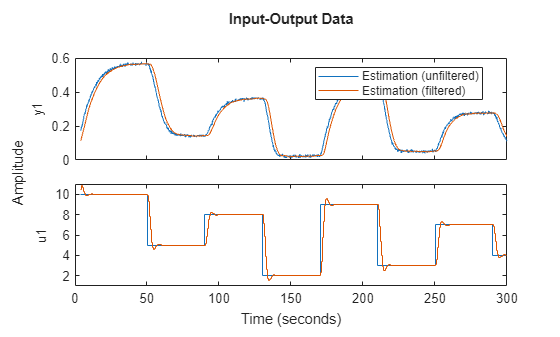# Machine-Learning-Based Identification of Two-Tank System

This example shows how to identify a black-box dynamic model of a nonlinear two-tank system.For this system, the input $\mathit{u}\left(\mathit{t}\right)$ is the voltage [V] applied to a pump, which generates an inflow to the upper tank. Water flows through a hole in the bottom of the upper tank and fills the lower tank. The output $\mathit{y}\left(\mathit{t}\right)$ of the two-tank system is the liquid level [m] of the lower tank.

The example uses measured data that contains 3000 input-output samples of a two-tank system generated using a sample time of 0.2 seconds. Load the data set and create an `iddata` object.

```load twotankdata z1f = iddata(y,u,0.2,'Name','Two-tank system');```

This data set is also used in the following examples.

This example is about black-box modeling using nonlinear ARX models with a regression tree ensemble model. Therefore, no knowledge of the actual physical laws is assumed.

Regression tree ensemble models are nonparametric models that are structured as a weighted combination of multiple regression trees. Each regression tree consists of decision nodes and leaf nodes. A decision node represents a value for an attribute (feature) being tested. A leaf node represents a numerical value associated with the decision . Regression models are estimated recursively by splitting the data set into progressively smaller components. Combining multiple regression trees improves the predictive performance of regression tree ensemble models.

### Preprocess Data

Before estimating a model, preprocess the data by first splitting it into estimation and validation data. Then, filter the data to remove high-frequency noise.

Split the data set into two subsets of equal size. You estimate the model using data set `z1` and validate the model using data set `z2`.

```z1 = z1f(1:1500); z2 = z1f(1501:3000); plot(z1,z2) legend('Estimation','Validation');```From the plot, you can see that the output data has high-frequency noise. Apply a low-pass filter to remove this noise.

```z1f = idfilt(z1,3,0.066902); z2f = idfilt(z2,3,0.066902);```

Remove the effect of the filter initial conditions from the data by trimming the initial data points from each data set.

```z1f = z1f(20:end); z2f = z2f(20:end);```

Plot the raw estimation data and the corresponding filtered data

```plot(z1(20:end),z1f) legend('Estimation (unfiltered)','Estimation (filtered)',... 'Location','best')```Plot the raw validation data and the corresponding filtered data

```plot(z2(20:end),z2f) legend('Validation (unfiltered)','Validation (filtered)',... 'Location','best') ```### Estimate and Validate Model

Create a decision tree ensemble mapping object with default settings. You can estimate nonlinear ARX models that use this nonlinearity as an output function. Using this mapping object requires Statistics and Machine Learning Toolbox™ software.

`ens = idTreeEnsemble;`

Estimate a nonlinear ARX model with both input and output regressors.

`mdl1 = nlarx(z1f,[30 30 0],ens);`

Estimate a nonlinear ARX model with input regressors only.

`mdl2 = nlarx(z1f,[0 150 0],ens);`

Compare the outputs of the estimated models with the measured estimation data.

`compare(z1f,mdl1,mdl2)`Compare the outputs of the estimated models with the measured validation data.

`compare(z2f,mdl1,mdl2)`